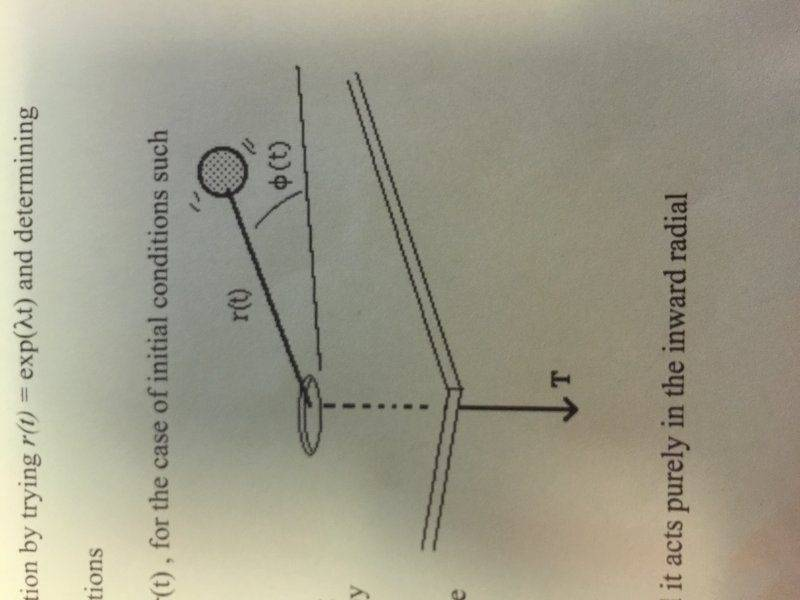# Determining the tension on a rotating particle

• freddie711
In summary, the homework statement is that a particle slides on a smooth table and is pulled downwards by a force. The equation for the tension is found by using F=ma in polar coordinates. The acceleration in the ##\small \theta## direction is found to be not equal to ##\ddot{\theta}##.

## Homework Statement

[/B]
A particle of mass m slides (both sideways and radially) on a smooth frictionless horizontal table. It is attached to a cord that is being pulled downwards at a prescribed constant speed v by a force T (T may be varying)

Use F=ma in polar coordinates to derive an expression for the tension T (T will depend on r and θ and how they may be changing)

Show the particle's polar coordinates satisfy r2dθ/dt = constant

HINT: The only horizontal force on the particle is T and it acts purely in the inward radial direction. Also, dr/dt is known and it is equal to -v

## Homework Equations

a = (r''(t)w2)r_hat +(r(t)θ''(t) + 2r'(t)θ'(t))θ_hat
L(t) = L_o ?

## The Attempt at a Solution

Not entirely sure how to get started. I've identified that a_r must be zero (since dr/dt is a constant, r''(t) must be zero making a_r zero). I've also set up the equation a_θ = r(t)θ''(t) - 2vw since r'(t) = v and θ'(t) = w. The issue I'm having is trying to identify another equation for the acceleration (or force) in the θ direction, and then from there rectifying that into T.

Welcome to PF!

If the force has only a radial component, what can you say about the component of acceleration in the ##\small \theta## direction: ## a_{\theta}##?

What is the expression in polar coordinates for ##a_{\theta}##?

That's part that's confusing... if there's only a force radially, θ''(t) should be zero. However, intuitively, as the radius gets shorter the particle should start rotating faster (cons. of ang. mom.) As far as aθ goes, I'm not exactly sure what the expression should be because I can't identify any forces working on the particle along that axis.

The other part that's messing with my head is the fact that, despite there being a force in the radial direction, there is no radial acceleration.

I've attached the image that came with the problem.freddie711 said:
That's part that's confusing... if there's only a force radially, θ''(t) should be zero.
It turns out that ##a_\theta## is not equal to ##\small \ddot{\theta}##. Note: From your first post you wrote a = (r''(t)w2)r_hat +(r(t)θ''(t) + 2r'(t)θ'(t))θ_hat. So, you can read off ##a_\theta## from there.

Last edited:
Oh. That makes a lot of sense, thanks. How would I use that to get to T(t) though?

freddie711 said:
How would I use that to get to T(t) though?